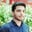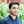Trusted answers to developer questions
Trusted Answers to Developer Questions

Related Tags

r
communitycreator

# What is the mean() method in R?Salman Yousaf

Grokking Modern System Design Interview for Engineers & Managers

Ace your System Design Interview and take your career to the next level. Learn to handle the design of applications like Netflix, Quora, Facebook, Uber, and many more in a 45-min interview. Learn the RESHADED framework for architecting web-scale applications by determining requirements, constraints, and assumptions before diving into a step-by-step design process.

The R programming language is used to perform statistical analysis. We can use built-in functions to accomplish our tasks. The mean() method is used to compute the arithmetic mean of the argument vector x. We can use the mean() method either column-wise or row-wise, depending on the logic of the program.

The mean is calculated by dividing the sum of all values by the total number of values in the dataset.

### Syntax

The syntax of the mean() function to calculate the average of values of x is as follows:

mean(x, trim = 0, na.rm = FALSE,...)


### Parameters

• x: A vector of numeric, logical, date, or date-time values. For complex vectors, trim should be zero.
• trim: Used to drop values from both sides of vector x before computing the mean. The default value is 0.
• na.rm: A boolean field that can be set to TRUE to remove NA(Not Available) values before computing the mean. The default value is False.

### Return value

mean() returns a numeric or complex vector with length one or NA_real_.

Calculating the mean of table data

### Code

In the code snippet below, we calculate the mean of vector x in line 5. In line 6, we return the return type.

# Create a vector.
x <- c(6,14,6,14.2,18,2,63,-21,9,-5,-4)
# Evaluate mean of numbers
mean_value <-  mean(x,trim = 0)
typeof(mean_value)
# Printing results
cat("Mean is:", mean_value)

The demo code below also calculates the mean of the vector x. trim = 0.2, which means it will drop two values (6,14) from the left and two values (-5,-4) from the right. Then mean() method will evaluate the arithmetic mean of the remaining values, (6,14.2,18,2,63,-21,9).

# Create a vector.x <- c(6,14,6,14.2,18,2,63,-21,9,-5,-4)# Find Mean with trim = 0.2mean_value <-  mean(x,trim = 0.2)cat("Trimed Mean: ", mean_value)

In line 2, we have a vector of numeric values containing an NA value. The mean() method in line 4 will return NA. So, in line 7, we set na.rm= TRUE, which removes NA values, and compute the mean of the remaining values.

# Create a vector. x <- c(6,14,6,14.2,18,NA,2,63,-21,9,-5,-4)# Find mean.mean_value <-  mean(x)cat("Wrong Output before: ", mean_value)# Find mean dropping NA values.mean_value <-  mean(x,na.rm = TRUE)cat("\nAfter removing : ", mean_value)

RELATED TAGS

r
communitycreator

CONTRIBUTORSalman Yousaf

Grokking Modern System Design Interview for Engineers & Managers

Ace your System Design Interview and take your career to the next level. Learn to handle the design of applications like Netflix, Quora, Facebook, Uber, and many more in a 45-min interview. Learn the RESHADED framework for architecting web-scale applications by determining requirements, constraints, and assumptions before diving into a step-by-step design process.

Keep Exploring

Learn in-demand tech skills in half the time